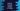# How to truncate number to integer in python## How to truncate number to integer in python:

In this post, we will learn how to truncate one number to integer in Python. For example, we can convert 13.1111 to 13. Python provides math.trunc and int() methods to truncate numbers.

In this post, I will show you how to use math.trunc and int() with examples.

### Example of math.trunc :

trunc() method is defined in math package in python. This method returns the truncated integer part of a given number. It will not round the number, but only returns the truncated integer value.

Below program shows how we can use math.trunc and results with different input values:

``````import math

print(math.trunc(1.13))
print(math.trunc(1.13889878999))
print(math.trunc(1.63))
print(math.trunc(-0.9989))
print(math.trunc(-0.9))
print(math.trunc(0.99898888788))
print(math.trunc(0.89))``````

It will print:

``````1
1
1
0
0
0
0``````

### Using int():

This is defined as below :

``int(strValue, base = 10)``

It returns one integer value. The base is optional. By default, it takes 10.

Let’s try it with the same inputs we used above:

``````print(int(1.13))
print(int(1.13889878999))
print(int(1.63))
print(int(-0.9989))
print(int(-0.9))
print(int(0.99898888788))
print(int(0.89))``````

It will print:

``````1
1
1
0
0
0
0``````